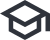### Here you can learn how to use it.

Note: These tutorials are in beta and may be subject to changes. If you find mistakes or have suggestions for improvements, let us know.

## Layout of the Tutorials (click to open)

Hyperlinks in the tutorials are mostly used to link technical terms to their corresponding Wikipedia article.

Buttons with the iconopen tutorials.

The iconindicates interactive tutorials that make use of our quantum circuit simulator.

Important results & statements in the main text are highlighted by yellow boxes:

Quantum mechanics is a fascinating topic!

The right page column is used for figures that accompany the text in the main column.

In addition, there are three types of textboxes:

Boxes with a yellow border contain brief summaries of the corresponding section in bullet point form.

Boxes with a red border contain important side notes to avoid misconceptions or answer anticipated questions.

Boxes with a green border contain optional side notes to provide additional context or examples.

### The Basics of Quantum Mechanics

In this tutorial you can learn what a qubit is, why qubits can be in superposition states, where the randomness in quantum mechanics enters, and why quantum computers can do things that classical computers cannot.

This tutorial provides the necessary knowledge to understand all other tutorials and to use our interactive quantum circuit simulator to run simple quantum algorithms on our servers.

### Quantum Algorithms & Quantum Circuits

In this tutorial you can learn how quantum algorithms can be represented as quantum circuits and how our interactive quantum circuit simulator can be used to run quantum algorithms on our servers.

### Superpositions, Randomness & Interference

In this tutorial you can learn how superpositions can be created in a quantum circuit, how randomness affects quantum computations, and how interference can be detected in measurement results.

### Coherence and Decoherence

Useful qubits must remain coherent for as long as possible. The goal of this tutorial is to understand what this means. You can learn how one can check whether a qubit remained coherent, and how the process of decoherence can be modeled with our quantum circuit simulator.

### Entanglement

The phenomenon of entanglement is perhaps the most prominent feature of quantum mechanics. In this tutorial you can learn how this can be done with the quantum circuit simulator and how entanglement is reflected in the measurement outcomes.

### Schrödinger’s cat

Schrödinger’s cat is a thought experiment to illustrate the strange implications that the predicted phenomena of superposition and entanglement can have. In this tutorial you recreate the basic mechanism of the thought experiment as a quantum circuit. We discuss why the experiment can never work in the real world.